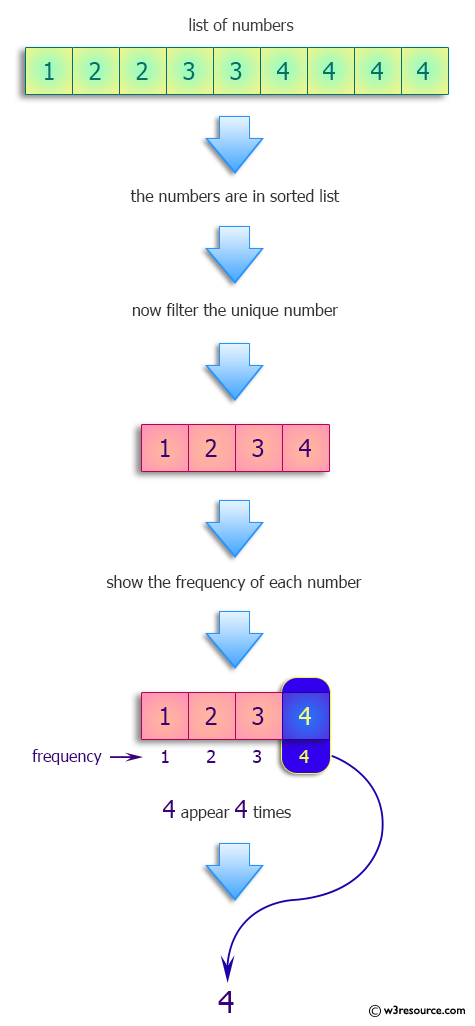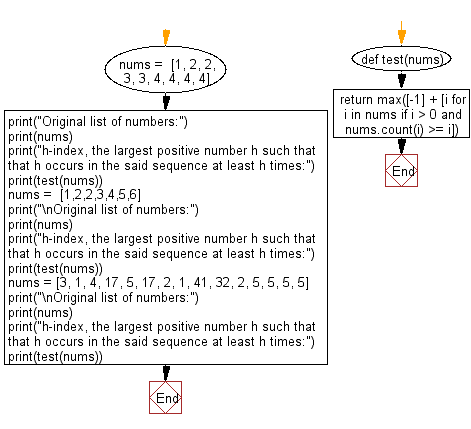﻿ Python: Find the h-index, the largest positive number h such that h occurs in the sequence at least h times - w3resource# Python: Find the h-index, the largest positive number h such that h occurs in the sequence at least h times

## Python Programming Puzzles: Exercise-49 with Solution

Write a Python program to find the h-index, the largest positive number h such that h occurs in the sequence at least h times. If there is no such positive number return h = -1.

```Input:
[1, 2, 2, 3, 3, 4, 4, 4, 4]
Output:
4

Input:
[1, 2, 2, 3, 4, 5, 6]
Output:
2

Input:
[3, 1, 4, 17, 5, 17, 2, 1, 41, 32, 2, 5, 5, 5, 5]
Output:
5
```

Pictorial Presentation:Sample Solution:

Python Code:

``````#License: https://bit.ly/3oLErEI

def test(nums):
return max([-1] + [i for i in nums if i > 0 and nums.count(i) >= i])

nums =  [1, 2, 2, 3, 3, 4, 4, 4, 4]
print("Original list of numbers:")
print(nums)
print("h-index, the largest positive number h such that h occurs in the said sequence at least h times:")
print(test(nums))
nums =  [1,2,2,3,4,5,6]
print("\nOriginal list of numbers:")
print(nums)
print("h-index, the largest positive number h such that h occurs in the said sequence at least h times:")
print(test(nums))
nums = [3, 1, 4, 17, 5, 17, 2, 1, 41, 32, 2, 5, 5, 5, 5]
print("\nOriginal list of numbers:")
print(nums)
print("h-index, the largest positive number h such that h occurs in the said sequence at least h times:")
print(test(nums))
``````

Sample Output:

```Original list of numbers:
[1, 2, 2, 3, 3, 4, 4, 4, 4]
h-index, the largest positive number h such that h occurs in the said sequence at least h times:
4

Original list of numbers:
[1, 2, 2, 3, 4, 5, 6]
h-index, the largest positive number h such that h occurs in the said sequence at least h times:
2

Original list of numbers:
[3, 1, 4, 17, 5, 17, 2, 1, 41, 32, 2, 5, 5, 5, 5]
h-index, the largest positive number h such that h occurs in the said sequence at least h times:
5
```

Flowchart:## Visualize Python code execution:

The following tool visualize what the computer is doing step-by-step as it executes the said program:

Python Code Editor :

Have another way to solve this solution? Contribute your code (and comments) through Disqus.

What is the difficulty level of this exercise?

Test your Programming skills with w3resource's quiz.

﻿

## Python: Tips of the Day

Clamps num within the inclusive range specified by the boundary values x and y:

Example:

```def tips_clamp_num(num,x,y):
return max(min(num, max(x, y)), min(x, y))
print(tips_clamp_num(2, 4, 6))
print(tips_clamp_num(1, -1, -6))
```

Output:

```4
-1
```